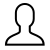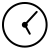# Lost in translation? Working with Matlab and PythonRobert (Bob) Turner14 October 2019 09:00

A huge amount of research code has been written in Matlab (Matrix laboratory), a paid-for product from Mathworks, and the Research Software Engineering (RSE) team here at the University of Sheffield have recently had a few enquires about either getting some of this code to work with Python or to translate it into Python altogether. I’m going to talk a bit here about the motivation for this and the technical strategies that we’re thinking about using. To be clear up-front, this is not a Matlab vs. Python tech-off. It mostly applies equally to if one were doing this the other way round.

Both Matlab and Python see a lot of use in universities. In my experience, Matlab is particularly popular with physicists and engineers. It has been taught to undergraduates and powerful toolboxes mean that it’s possible to put together complex data analysis and simulation workflows with minimal coding. Python is increasingly being taught at an undergraduate level - as a general purpose language it’s in some ways more versatile than Matlab which is really geared up for scientific and engineering applications. Python sees use in bioinformatics.

## If it ain’t broke…

If you’ve got an application in Matlab, surely you can keep using, modifying and adding to it? No? Reasons?

These reasons are all about scaling up (which is something a lot of research has to address when moving from a lab/academic context, not just software):

• I can’t afford to keep paying for the license.
• My target users don’t have a license.
• I want to deploy my application in a container (let’s say Docker) and I don’t have a license to do this.

These reasons are all about responding to new requirements:

• I want to use a library/package/toolbox that’s only available in a different language.
• I want to use a language feature that’s only available in a different language.

There are plenty of instances where sticking with Matlab is absolutely the right call. And at no point is anyone saying that something shouldn’t have been done in a certain way!

## Let’s “fix” it…

So what are our options? Here goes:

### Re-write all of the Matlab code in Python

This is going to take a while depending on how much code there is. The first question here is: How much are we relying on Matlab toolboxes? These can do a lot of things that are not in base Python. One can find out what toolboxes are used by a group of .m files using:

``````[dfiles,products]=matlab.codetools.requiredFilesAndProducts(mfiles);
``````

Where `products` is the list of toolboxes. This is a starting point to begin finding alternatives - e.g. scikit-image has some of the functionality of Matlab’s Image Processing Toolbox. Anything that is not available may need to be built from scratch. It may be a good idea to carefully scope the project to ensure that the requirements of the new application are tightly defined. If the old application does stuff that’s not required in the new one, that baggage can be left behind. Another consideration is whether to refactor / reorganise the Matlab code, depending on how it’s been put together. If it’s, for example, one continuous script lacking functions, it may be a good idea to organise it such that a 1:1 map can then be made between functions in the two languages. However, doing this might mean losing out on Python language features that are not available (or not generally used) in Matlab. To highlight these differences: Matlab leans heavily towards arrays, a variable type that is not natively supported in Python (one can, of course, use numpy).

### Call Matlab code from Python (e.g. if we want to integrate our Matlab functionality into a new application)

If we decide we don’t fancy completely re-doing our project, other options are available. One is to call Matlab code from within Python using the Matlab Engine for Python. We can do this kind of thing:

``````import matlab.engine
eng = matlab.engine.start_matlab()
mla = eng.rand(1e3,'double')
mla_t = eng.transpose(mla)
``````

In which we make and transpose a Matlab array, using Python. However, if we try to change a Python (numpy) array into a Matlab array, we can’t:

``````matlab_array=eng.double(numpy_array) #fails
``````

This may be a bit limiting - here’s a list of what’s allowed. However, there are a couple of potential ways around this - see this post. Or…

### Call Python code from Matlab (e.g. if we want to use new Python functionality that’s not available in Matlab)

We can call Python from Matlab like this, assuming it’s installed correctly:

``````my_numpy_array=py.numpy.random.rand(int8(1e4),int8(1e4));
``````

Here I’ve made a `numpy.array`. Good for me. And in this context, I can turn it into a Matlab array:

``````my_matlab_array=double(my_numpy_array);
``````

This opens up the possibility of accessing Python functionality while leaving most of the code in Matlab. Some more detail on this can be found here.

## Now what?

The best approach is going to be very project-dependant, and may entail a mixture of what I’ve talked about, above. The RSE team can offer a range of levels of support for this from code clinics through to complete translation between languages.

To summon the RSE team, project a silhouette of the Git logo onto the clouds above Sheffield. Or visit the Sheffield RSE Website.